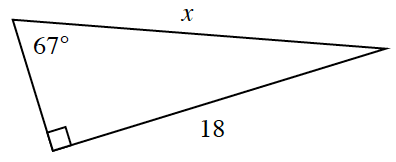### Home > INT2 > Chapter 4 > Lesson 4.2.4 > Problem4-95

4-95.Estelle is trying to solve for $x$ in the triangle at right. She lost her scientific calculator, but luckily her teacher tells her that $\sin23^\circ\approx0.391$, $\cos23^\circ\approx0.921$, and $\tan23^\circ\approx0.424$.

1. Write an equation that Estelle could use to solve for $x$.

Recall: $\text{sin }(\theta)=\frac{\text{opp.}}{\text{hyp.}}$ and $\cos(\theta)=\frac{\text{adj.}}{\text{hyp.}}$

2. Without a calculator, how could Estelle determine sin $67^\circ$? Explain.

What angle is complementary to $67^\circ$?